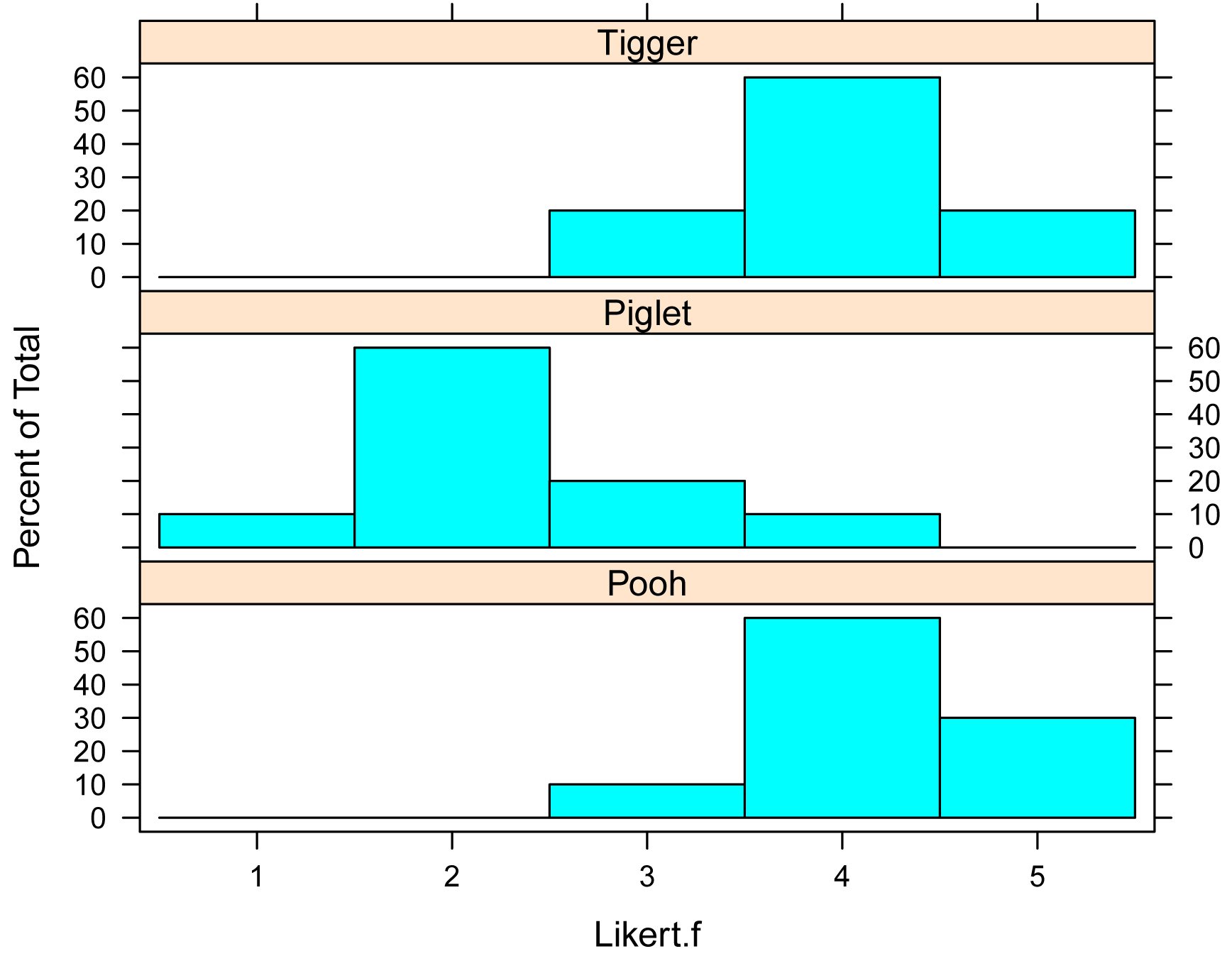## Summary and Analysis of Extension Program Evaluation in R

Salvatore S. Mangiafico

# One-way Ordinal Regression with CLM

##### Appropriate data

•  One-way data with two or more groups

•  Dependent variable is ordered factor

•  Independent variable is a factor with at least two levels or groups

•  Observations between groups are not paired or repeated measures

##### Interpretation

A significant result can be interpreted as, “There was a significant difference among groups.”  Or, “There was a significant effect of Independent Variable.”

A significant post-hoc analysis indicates, “There was a significant difference between Group A and Group B”, and so on.

### Packages used in this chapter

The packages used in this chapter include:

•  psych

•  FSA

•  lattice

•  ordinal

•  car

•  RVAideMemoire

•  multcomp

•  emmeans

The following commands will install these packages if they are not already installed:

if(!require(psych)){install.packages("psych")}
if(!require(FSA)){install.packages("FSA")}

if(!require(lattice)){install.packages("lattice")}
if(!require(ordinal)){install.packages("ordinal")}
if(!require(car)){install.packages("car")}
if(!require(RVAideMemoire)){install.packages("RVAideMemoire")}
if(!require(multcomp)){install.packages("multcomp")}
if(!require(emmeans)){install.packages("emmeans")}

### One-way ordinal regression example

The following example revisits the Pooh, Piglet, and Tigger data from the Kruskal–Wallis Test chapter.

Speaker  Likert
Pooh      3
Pooh      5
Pooh      4
Pooh      4
Pooh      4
Pooh      4
Pooh      4
Pooh      4
Pooh      5
Pooh      5
Piglet    2
Piglet    4
Piglet    2
Piglet    2
Piglet    1
Piglet    2
Piglet    3
Piglet    2
Piglet    2
Piglet    3
Tigger    4
Tigger    4
Tigger    4
Tigger    4
Tigger    5
Tigger    3
Tigger    5
Tigger    4
Tigger    4
Tigger    3
")

### Order levels of the factor; otherwise R will alphabetize them

Data\$Speaker = factor(Data\$Speaker,
levels=unique(Data\$Speaker))

### Create a new variable which is the likert scores as an ordered factor

Data\$Likert.f = factor(Data\$Likert,
ordered = TRUE)

###  Check the data frame

library(psych)

str(Data)

summary(Data)

#### Summarize data treating Likert scores as factors

xtabs( ~ Speaker + Likert.f,
data = Data)

Likert.f
Speaker  1 2 3 4 5
Pooh   0 0 1 6 3
Piglet 1 6 2 1 0
Tigger 0 0 2 6 2

XT = xtabs( ~ Speaker + Likert.f,
data = Data)

prop.table(XT,
margin = 1)

Likert.f
Speaker    1   2   3   4   5
Pooh   0.0 0.0 0.1 0.6 0.3
Piglet 0.1 0.6 0.2 0.1 0.0
Tigger 0.0 0.0 0.2 0.6 0.2

#### Bar plots by group

library(lattice)

histogram(~ Likert.f | Speaker,
data=Data,
layout=c(1,3)      #  columns and rows of individual plots
)#### Summarize data treating Likert scores as numeric

library(FSA)

Summarize(Likert ~ Speaker,
data=Data,
digits=3)

Speaker  n mean    sd min Q1 median   Q3 max percZero
1    Pooh 10  4.2 0.632   3  4      4 4.75   5        0
2  Piglet 10  2.3 0.823   1  2      2 2.75   4        0
3  Tigger 10  4.0 0.667   3  4      4 4.00   5        0

#### One-way ordinal regression

The model is specified using formula notation.  Here, Likert.f is the dependent variable and Speaker is the independent variable.  The data= option indicates the data frame that contains the variables.  For the meaning of other options, see ?clm.

##### Define model

library(ordinal)

model = clm(Likert.f ~ Speaker,
data = Data)

##### Analysis of deviance

anova(model, type="II")

Type II Analysis of Deviance Table with Wald chi-square tests

Df  Chisq Pr(>Chisq)
Speaker  2 13.498   0.001172 **

##### Comparison of models analysis

model.null = clm(Likert.f ~ 1, data=Data)

anova(model, model.null)

Likelihood ratio tests of cumulative link models:

no.par    AIC  logLik LR.stat df Pr(>Chisq)
model.null      4 91.693 -41.847
model           6 72.298 -30.149  23.395  2  8.315e-06 ***

### The p-value is for the effect of Speaker

##### Alternate analysis of deviance analysis

library(car)

library(RVAideMemoire)

Anova.clm(model, type = "II")

Analysis of Deviance Table (Type II tests)

LR Chisq Df Pr(>Chisq)
Speaker   23.395  2  8.315e-06 ***

##### Post-hoc test with emmeans

In the emmeans function, model specifies the model object that was previously fitted.  Note the specialized formula where pairwise indicates that all pairwise comparisons should be conducted, and Instructor indicates the variable whose levels will be compared.

For clm model objects, the emmean, SE, LCL, and UCL values should be ignored, unless specific options in emmeans are selected.

library(emmeans)

marginal = emmeans(model,
~ Speaker)

pairs(marginal,

contrast        estimate    SE  df z.ratio p.value
Pooh - Piglet      4.944 1.376 Inf   3.592  0.0010
Pooh - Tigger      0.634 0.906 Inf   0.700  0.7636
Piglet - Tigger   -4.310 1.317 Inf  -3.272  0.0031

P value adjustment: tukey method for comparing a family of 3 estimates

library(multcomp)

cld(marginal, Letters=letters)

Speaker emmean    SE  df asymp.LCL asymp.UCL .group
Piglet   -1.78 0.776 Inf    -3.304    -0.263  a
Tigger    2.53 0.841 Inf     0.878     4.175   b
Pooh      3.16 0.891 Inf     1.413     4.907   b

Confidence level used: 0.95
P value adjustment: tukey method for comparing a family of 3 estimates
significance level used: alpha = 0.05

NOTE: Compact letter displays can be misleading
because they show NON-findings rather than findings.
Consider using 'pairs()', 'pwpp()', or 'pwpm()' instead.

### Groups sharing a letter in .group are not significantly different

### Remember to ignore “emmean”, “SE”, “LCL”, and “UCL” for CLM

##### Check model assumptions

nominal_test(model)

Tests of nominal effects

formula: Likert.f ~ Speaker
Df  logLik    AIC LRT Pr(>Chi)
<none>     -30.149 72.298
Speaker

###  No p-value

scale_test(model)

Tests of scale effects

formula: Likert.f ~ Speaker
Df  logLik    AIC     LRT Pr(>Chi)
<none>     -30.149 72.298
Speaker  2 -29.839 75.677 0.62096   0.7331

###  No violation in assumptions

#### Effect size statistics

Appropriate effect size statistics may include those appropriate for the Kruskal–Wallis test.